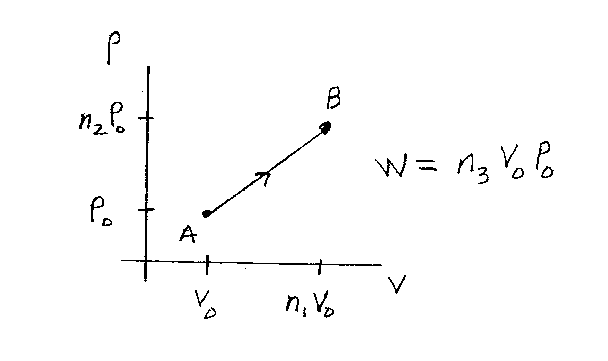Problem D8: Consider an ideal gas that is confined to a container. The gas starts in the state A. The gas then undergoes a quasi-static process to the state B that is shown in the figure below. In state A the volume is V0 and the pressure is P0. In state B the volume of the gas is n1V0 and the pressure is n2P0. The process is described by a straight line in the P-V plane. If the work done by the gas is W = n3 P0V0, what is n3? Note that n1, n2 and n3 are unitless.n1 = n2 = Input n3:

If you are currently in my class, you can record your grade by entering your name and student ID number (without the leading zeros) below and clicking on "record grade".
 First Name = Last Name = ID = Problem: